# Understanding Data Distributions

Travis Hartin, Mia Primas
• Author
Travis Hartin

Travis has taught college-level statistics, research methods, and psychology courses for eight years. Travis has a Master’s degree and PhD in experimental psychology from Kent State University with a focus on student learning and cognitive research.

• Instructor
Mia Primas

Mia has taught math and science and has a Master's Degree in Secondary Teaching.

Learn what is data distribution. Study about different data distribution types, their shapes & characteristics. Learn how to find the distribution of a data set. Updated: 12/25/2021

Show

## What is Data Distribution?

Researchers that collect data during studies often find themselves with large sets of data that they need to simplify in order for them to communicate their findings to different audiences. To do this, they often use what is called a data distribution. A data distribution is a graphical representation of data that was collected from a sample or population. It is used to organize and disseminate large amounts of information in a way that is meaningful and simple for audiences to digest. For example: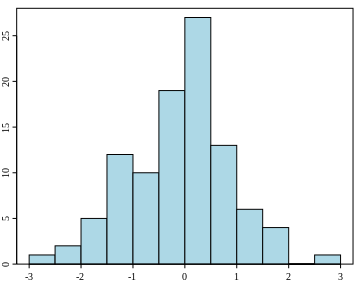Figure 1 is an example of a histogram. It displays the frequency in which different values or outcomes are observed in a particular sample. It is also referred to as a frequency distribution. The horizontal line (i.e., the x-axis) represents the different values that could occur during a study and the vertical line (i.e., the y-axis) represents the frequency that each value was observed. The shape of the distribution is also informative. The peak of the graph represents the most frequent values and the smaller tails on both sides of the peak represent the least common values. Instead of looking for trends in a database of unorganized numbers, researchers use histograms as a way to communicate their findings.

Histograms are used to show frequencies of large sets of data.

Here is another example of a method used to represent a distribution of data: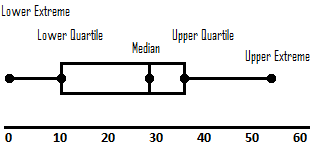Figure 2 is an example of a box plot. They are also called box-and-whisker plots. They are used to summarize a few key statistics of a sample of data:

• Quartile: This is a value that divides the number of data points in a set of data in four equal parts or quarters. In a box plot, the bar represents the middle 50% of the data.
• Median: This is the middle score when all of the values in a distribution are organized from lowest to highest.
• Extreme: The extremes are the lowest and highest values in a set of data.

Similar to a histogram, box plots summarize key trends found in sets of data.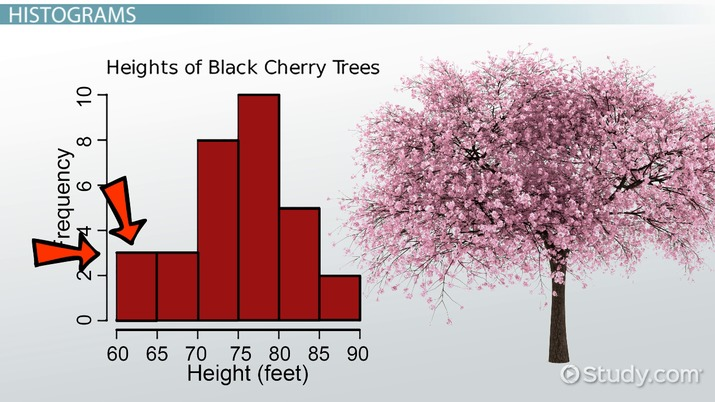An error occurred trying to load this video.

Try refreshing the page, or contact customer support.

Coming up next: Application of Statistics in Daily Life

### You're on a roll. Keep up the good work!

Replay
Your next lesson will play in 10 seconds
• 0:00 What is Data Distribution?
• 0:40 Dot Plots
• 1:25 Histograms
• 2:01 Box Plot
• 3:12 Tally Charts
• 3:54 Lesson Summary
Save Save

Want to watch this again later?

Timeline
Autoplay
Autoplay
Speed Speed

## Data Distribution Types

There are two different types of data in statistics: discrete data and continuous data.

#### Discrete Data

Discrete data involves variables that have specific values that cannot have values between them. For example, the number of times someone visits their neighbors during the week is a discrete variable. Someone can visit their neighbor 0, 1, 2, 3, or even 10 times during the week. However, someone cannot visit their neighbor 1.65 or 3.09 times. Categorical variables (e.g., genres of music, political parties) are also considered discrete variables because they involve clear categorical boundaries.

#### Continuous Data

Continuous data involves variables in which there could be an infinite number of values between a set range of possible values. For example, students can theoretically score an infinite number of final exam grades on a scale of 0 to 100. Someone could score a 90, 90.01, 90.324, 90.993, 89.873, etc. Another example of a continuous variable are things measured in time. A 26-year-old might express their age as 26, 26.25, or 26.2534 depending on their level of specificity.

There are also three different types of data distribution based on the distribution of values in the data.

#### Symmetrical Distribution

A symmetrical distribution is when the pattern or trend of frequencies on the left and right side of the distribution are the same.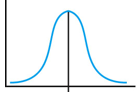#### Skewed Distribution

A skewed distribution is when the scores pile or stack up on one side and are spread out on the other (i.e., a distribution that is not symmetrical). There are two types of skewed distributions:

• Positive Skew: This is when the scores pile up on the lower end of the values with fewer scores at the high end. The side with fewer scores is called the tail and is considered the direction of the skew. See Figure 4 for an example.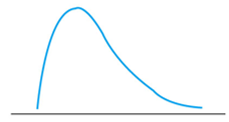Notice how the side with fewer scores is more spread out and looks like a tail. Since the tail is towards the higher end of the values, it is called a positive skew (i.e., it is skewed right because the tail is pointing to the right).

• Negative Skew: This is when the scores pile up on the higher end of the values with fewer scores at the low end. See Figure 5 for an example.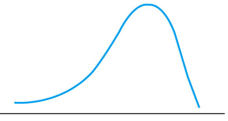Since the tail is towards the lower end of the values, it is called a negative skew (i.e., it is skewed left because the tail is pointing to the left).

### Discrete Data Distribution

There are different types of discrete data distributions that are used specifically for discrete data.

#### Binomial Distribution

A binomial distribution is used to represent the frequency of data that involves only two outcomes: passing and failing. Just think that "bi" means two and "nomial" means names or values. For example, a school district keeps track of students who pass or fail a particular high school course (e.g., AP Statistics). The only outcomes for this study are that the students either pass or fail. It is important to note that the variable in this example is discrete. Students cannot score a value in the middle of passing or failing.

Researchers often use parameters to describe patterns observed in a particular population. For binomial distributions, the parameters include:

• n = the number of data points (i.e., trials or pass/fail observations)
• p = the probability of success or passing

#### Poisson Distribution

A Poisson distribution is used to represent the frequency of something occurring during a specific time period. In other words, it counts how many times an event happens. For example, the previously mentioned school district keeps track of how many times students are absent during flu season. Notice that the variables in this example are also discrete. Students during this time period can be absent 0, 1, 2, 3, 4, times etc., but they cannot be absent 2.5, 3.76, or 0.154 times.

The Poisson distribution uses the rate parameter. This is essentially the likelihood that an event is expected to occur across a specific time period (e.g., a rate of 19 school absences during flu season).

To unlock this lesson you must be a Study.com Member.

#### What are the different types of data distribution?

There are two types of data distribution based on two different kinds of data: Discrete and Continuous. Discrete data distributions include binomial distributions, Poisson distributions, and geometric distributions. Continuous data distributions include normal distributions and the Student's t-distribution.

#### How do you find the distribution of data?

A probability plot is used to determine the distribution of data. It is a test that graphs data points along a straight line. Data that fit along that line qualify as that given type of distribution.

### Register to view this lesson

Are you a student or a teacher?